# dynamic Bayesian networks

In :

import pyAgrum as gum
import pyAgrum.lib.notebook as gnb
import pyAgrum.lib.dynamicBN as gdyn


## Building a 2TBN

Note the naming convention for a 2TBN : a variable with a name $$A$$ is present at t=0 with the name $$A0$$ and at time t as $$At$$.

In :

#twodbn=gum.BayesNet()
#                   for s in ["a0","b0","c0","at","bt","ct"]]
#       for s in ["d0","dt"]]

#twodbn.generateCPTs()
twodbn=gum.fastBN("d0->ct<-at<-a0->b0->bt<-a0->dt<-d0<-c0->ct;c0->at",6)
twodbn

Out:


### 2TBN

The dbn above actually is a 2TBN and is not correctly shown as a BN. Using the naming convention, it can be shown as a 2TBN.

In :

gdyn.showTimeSlices(twodbn)### unrolling 2TBN

A dBN is ‘unrolled’ using the 2TBN and the time period size. For a couple $$a_0$$,$$a_t$$ in the 2TBN, the unrolled dBN will include $$a_0, a_1, \cdots, a_{T-1}$$

In :

T=5

dbn=gdyn.unroll2TBN(twodbn,T)
gdyn.showTimeSlices(dbn,size="10")We can infer on bn just as on a normal bn. Following the naming convention in 2TBN, the variables in a dbN are named using the convention $$a_i$$ where $$i$$ is the number of their time slice.

In :

gnb.flow.clear()
for i in range(T):
gnb.flow.add_html(gnb.getPosterior(dbn,target="d{}".format(i),evs={}),"$P(d{})$".format(i))
gnb.flow.display()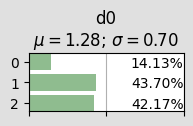$P(d0)$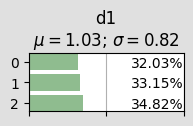$P(d1)$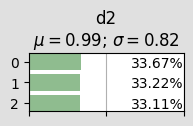$P(d2)$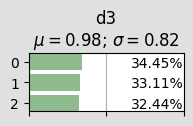$P(d3)$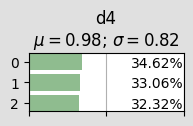$P(d4)$

### dynamic inference : following variables

gdyn.plotFollow directly ask for the 2TBN, unroll it and add evidence evs. Then it shows the dynamic of variable $$a$$ for instance by plotting $$a_0,a_1,\cdots,a_{T-1}$$.

In :

import matplotlib.pyplot as plt

plt.rcParams['figure.figsize'] = (10, 2)
gdyn.plotFollow(["a","b","c","d"],twodbn,T=51,evs={'a9':2,'a30':0,'c14':0,'b40':0,'c50':3})### nsDBN (Non-Stationnary Dynamic Bayesian network)

In :

T=15

dbn=gdyn.unroll2TBN(twodbn,T)
gdyn.showTimeSlices(dbn)Non-stationnaty DBN allows to express that the dBN do not follow the same 2TBN during all steps. A unrolled dbn is a classical BayesNet and then can be changed as you want after unrolling.

In :

# new P(ct|c0)
pot.fillWith([1,0,0,0.1]*9).normalizeAsCPT() # 36 valeurs normalized as CPT

Out:

ct
c0
0
1
2
3
4
5
0
0.47620.00000.00000.04760.47620.0000
1
0.00000.08330.83330.00000.00000.0833
2
0.47620.00000.00000.04760.47620.0000
3
0.00000.08330.83330.00000.00000.0833
4
0.47620.00000.00000.04760.47620.0000
5
0.00000.08330.83330.00000.00000.0833
In :

# from steps 5 to 10, $C_t$ only depends on $C_{t-1}$ and follows this new CPT
for i in range(5,11):
dbn.eraseArc(f"d{i-1}",f"c{i}")
dbn.eraseArc(f"a{i}",f"c{i}")
dbn.cpt(f"c{i}").fillWith(pot,["ct","c0"]) # ct in pot <- first var of cpt, c0 in pot<-second var in cpt

gdyn.showTimeSlices(dbn,size="14")In :

plt.rcParams['figure.figsize'] = (10, 2)
gdyn.plotFollowUnrolled(["a","b","c","d"],dbn,T=15,evs={'a9':2,'c14':0})In [ ]: